# Physics - Classical Mechanics - Exercises around Newtonian Gravity (part 1)

in StemSocial17 days ago[Image1]

## Introduction

Hey it's a me again @drifter1!

In this article we will continue with Physics, and more specifically the branch of "Classical Mechanics". Today's article will be about Exercises on Newtonian Gravity, and splitted into two parts.

So, without further ado, let's get straight into it!

## Recap of Useful Formulas

The following formulas will be very useful for solving Problems around Gravity (in part 1).

### Universal Law of Gravitation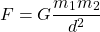where:
• d is the distance of the COMs of the two masses m1 and m2
• G is the Gravitation Constant which equals 6.674 × 10-11 N

### Weight or Force of Gravity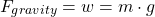### Gravitational Acceleration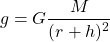where:
• r can be thought of as the median radius of a planet
• h as the height of an object in respect to the surface of the planet

## Gravitational Attraction of the Earth in Various Heights (based on Ref1 Sample Problems)

Let's consider a person of mass m = 60 Kg is inside the Gravitational Field of the Earth (which has a mass of M = 5.98 x 1024 Kg and radius of r = 6.38 x 106 m).

Calculate the Force of Gravity that the Earth exerts on the person when:

1. The person is standing at sea-level (distance to the center of the earth equal to the radius r)
2. The person is standing at the peak of Mount Everest (highest altitude above mean sea level is h = 8,848 m
3. The person is on an airplane that flies at about h = 12 km above sea-level
We simply have to apply the Universal Law of Gravitation for each case...

### 1. Sea-Level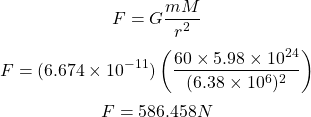### 2. Mount Everest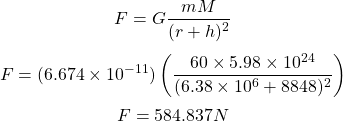### 3. Airplane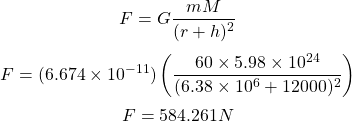The difference is minimal on so even using the value g = 9.8 m/s2 for Earth would be sufficient enough as it gives: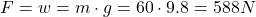## Calculating the Mass of the Earth

Knowing that the equatorial radius of the Earth is RE = 6.380 Km and the Gravitational acceleration is g = 9.8 m/s2, can we somehow calculate the mass of the Earth?

### Solution

Let's consider a test-mass of mass m = 1 Kg.

Solving the equation of gravitational acceleration g for the mass of the Earth ME we have: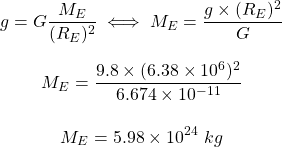## Multiple Attractors and Force of Gravity

### One-Dimensional Problem

Let's consider the following problem: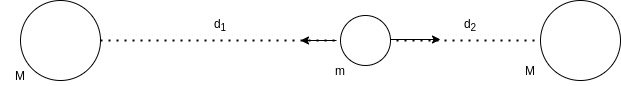[Custom Figure using draw.io]

Calculate the total force of gravity excelled on sphere m by the two spheres with equal mass M if d1 is greater than d2.

The result will be an expression of m, M, d1 and d2.

#### Solution

Because d1 > d2 we already know that F1 < F2.

Thus, the total force will be ΣF = F2 - F1.

Using the Universal Law of Gravitation we have: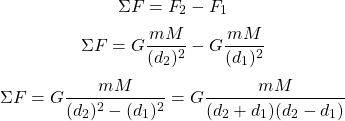The difference of squares a2 - b2 = (a + b)(a - b) is useful when more than 2 masses attract the object, as it simplifies the result by a lot.

### Two-Dimensional Problem

Let's consider the following problem: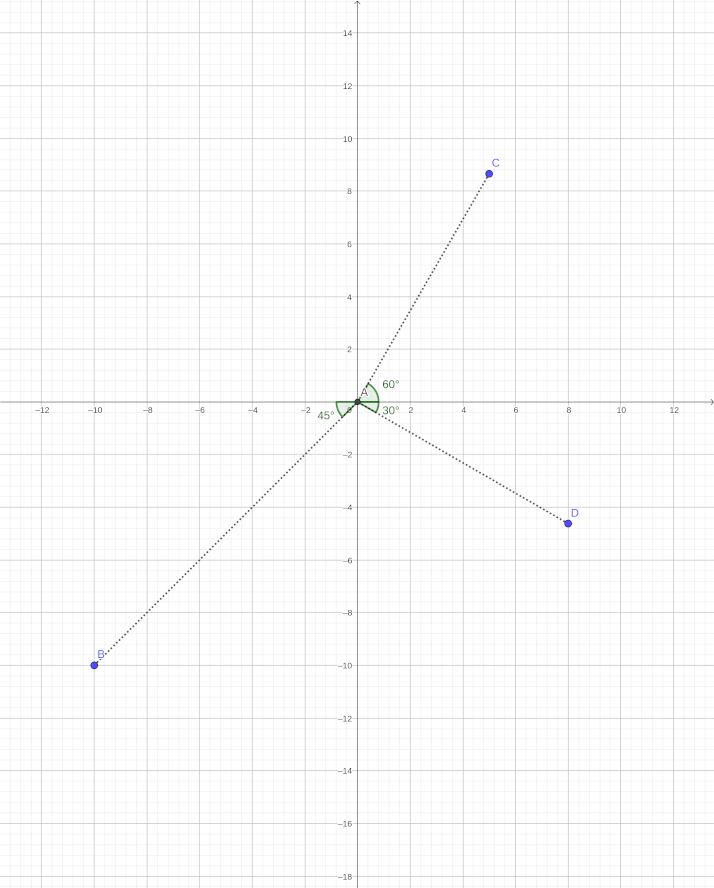[Custom Figure using GeoGebra]

In this figure, A (0, 0), B (-10, -10), C (5, 8.66) and D (8, -4.618) represent the centers of mass of the point masses: mA = 4 Kg, mB = 11 Kg, mC = 9 Kg and mD = 7 Kg.

The distance of mass A to each of the other masses is dAB = 14.14 m, dAC = 10 m and dAD = 9.24 m.

The Force of Gravitational Attraction from the masses B, C and D towards A, has an angle of β = 45°, γ = 60° and δ = 30° correspondingly, always in respect to the horizontal or x-axis.

Let's calculate the total Gravitational Force (ΣF) that attracts mass A.

#### Solution

Using the Universal Law of Gravitation we can calculate the force of attraction of each mass B, C and D towards A: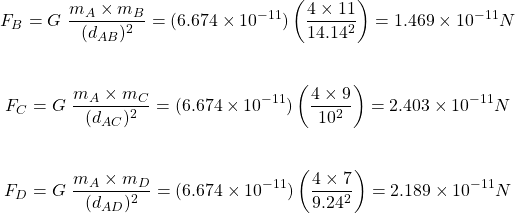In order to calculate the sum of those forces we have to split the forces into two components: one for the horizontal axis and one for the vertical axis.

The components are defined easily using Trigonometric Functions: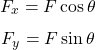In the case of the force FB which attracts mass A towards mass B we have the following components: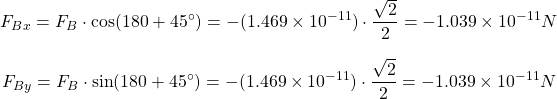(The results are negative because sin and cos have negative values in the 3rd Quadrant)

Similarly, for force FC from mass C we have: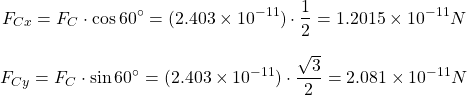Lastly, for force FD from mass D we have: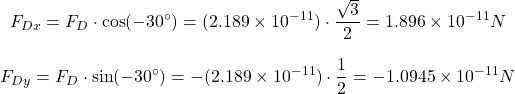(The trigonometric function sin is negative in the 4th Quadrant, whilst cos is positive)

The signs shows us where the vectors are pointing (left-right and up-down).

Now that we have the components we can easily calculate the components of ΣF: ΣFx and ΣFy as: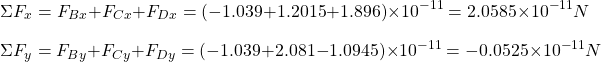Thus, the total force is: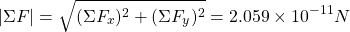and with an angle of: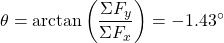## Final words | Next up

And this is actually it for today's post!

In part 2 we will get into exercises around Gravitational Fields and Gravitational Potential Energy...

See ya!Keep on drifting!
Sort:Thanks for your contribution to the STEMsocial community. Feel free to join us on discord to get to know the rest of us!

Please consider supporting our funding proposal, approving our witness (@stem.witness) or delegating to the @stemsocial account (for some ROI).

Please consider using the STEMsocial app app and including @stemsocial as a beneficiary to get a stronger support.